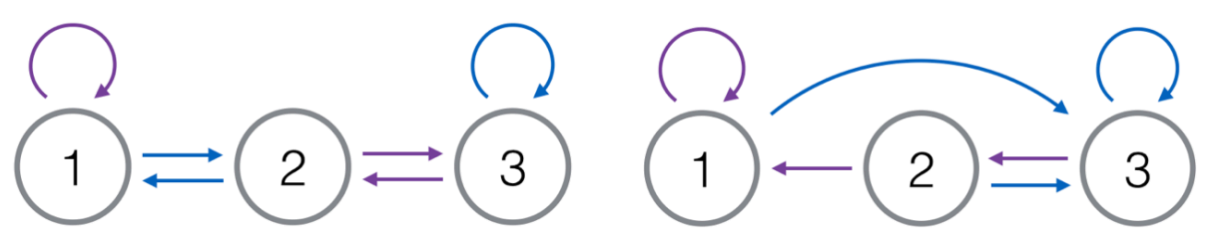# #375. 【ZJOI2018】迷宫

• 迷宫可以被抽象成 $n$ 个点，$nm$ 条边的有向图。$1$ 号点是唯一的入口也是唯一的出口。
• 每一个点恰好有 $m$ 条出边，且这些出边被依次标号为 $[0, m)$ 的正整数。
• 迷宫允许自环和重边。

### 样例一

#### input

3
2 3
2 4
6 8



#### output

3
3
5



#### explanation### 数据范围与约定

$m\ge 2$

1 $\le 6$ $\le 10$ $\le 100$
2 $\le 100$ $\le 100$ $\le 100$
3 $\le 100$ $\le 100$ $\le 100$
4 $\le 10^5$ $\le 10^5$ $\le 100$
5 $\le 10^5$ $\le 10^5$ $\le 100$
6 $\le 10^9$ $\le 10^9$ $\le 1000$ $m$为质数
7 $\le 10^9$ $\le 10^9$ $\le 1000$
8 $\le 10^9$ $\le 10^9$ $\le 1000$
9 $\le 10^{18}$ $\le 10^{18}$ $\le 3\times 10^5$
10 $\le 10^{18}$ $\le 10^{18}$ $\le 3\times 10^5$